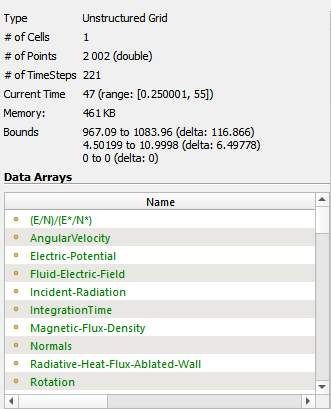# Plot/Calculate along a Streamlines

Dear Everybody,

my goal is to perform a calculation along a streamline:
I need to evaluate a certain integral along a streamline. The means following the streamline I would like to: Extract certain cell values, calculate a quotient of the variables, sum the calculated value for each individual cell over all cells that are crossed by the streamline. The final scalar value is the result that I am interested in.

I would appreciate any help or advice!!! What I need is a bit like plot along line and a python calculator combind. However, I would not even know what output type to specify, since the value of interest is a single scalar value…
So any help is appreciated a LOT. Some Ideas/Buzz words: extract cells along streamline as datasource, python calculator/programmable filter, output type, Integrate/sum, scalar value,

Many thanks to you all,
Ruben

P.S. I am dealing with an unstructured 2D grid. The cells are triangles of different size and shape.

Hi @Ruben

StreamTracer + PythonCalculator should do the trick.

I have problems with the datatypes that are generated. Also I am not 100 percent sure, how to access the field data within the python calculater. Do you have a good resource for self study? Because the VTK documentation did not really help me a lot…

From what I understand, the output type of Stremtracer are polylines? When I extract the data generated by the streamtracer, I end up with the following information on the data.Sorry I am a bit lost…
My physical fields consist of cell data and point data. To calculate the final value, I will have to access both, cell and point data (Temperature and Pressure → Cell, Electric Field → Point). The streamline is a streamline of the electric field.

So I understand right that the general idea is: Generate input data for the python calculator with the help of the streamtracer feature?

Many thanks and excuse my confusing reply,
Ruben

This output looks great ! You do not even need a PythonCalculator, the DescriptiveStatistics filter should do just what you need, you will find the sum in the DerivedStatistics block: